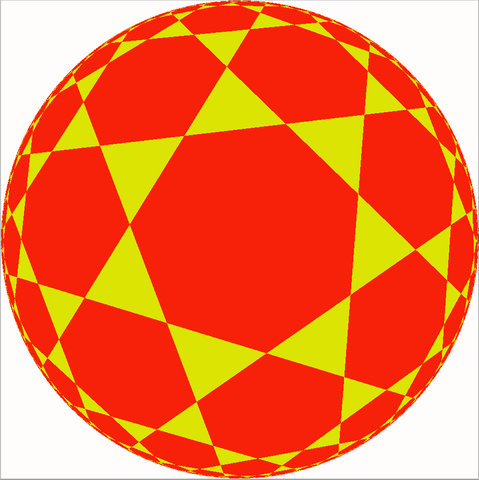You may have heard of the Poincaré disk model of the hyperbolic plane, in which the whole hyperbolic plane is mapped to the interior of a Euclidean disk, with hyperbolic lines being transformed into circular arcs that make a right angle with the disk boundary. Or of the Beltrami–Klein model, which also maps the hyperbolic plane to the interior of a disk, but with a different map that keeps the lines straight, so that they correspond to the chords of a circle.One use for these models is in information visualization, to provide a fisheye view of a drawing in the hyperbolic plane that provides "focus+context": focus on some specific feature in the drawing, and context of all the rest of the drawing, compressed into the outer parts of the disk. Another is as an aid to mathematical intuition: hyperbolic lines may be hard to visualize and understand, but chords of a circle are much more familiar, and this model shows that (in terms of the combinatorial patterns they can perform, if not their distances and angles) they are really the same thing.

But did you know that you can get an analogous fisheye view of the Euclidean plane, by another pair of models that map Euclidean lines to natural families of curves in a disk?

For any function $$f$$ that maps the ray $$[0,\infty)$$ one-to-one to an interval $$[0,r)$$ one can obtain a fisheye view of the Euclidean plane by mapping polar coordinates $$(\theta,r)$$ to $$(\theta,f(r))$$. But there are a couple of particularly nice choices that can be obtained geometrically, by a three-dimensional projection. Consider the plane as part of a three-dimensional space, with a sphere tangent to the plane at the point you want to focus on, and with the sphere's radius proportional to the size of the feature you want to focus on. Then there are three natural mappings between the sphere and the plane. For any point $$p$$ on the upper hemisphere of the sphere, there is a unique ray from the center of the sphere through $$p$$, and the point where this ray crosses the plane is the central projection of $$p$$. Similarly, there is a unique ray from the pole of the sphere (the point opposite its tangency with the plane) through $$p$$, and the point where this ray crosses the plane is the polar projection of $$p$$. And there is a unique line perpendicular to the plane through $$p$$, and the point where it crosses the plane is the perpendicular projection of $$p$$. Here they are in cross-section, giving the central, polar, and perpendicular projections between a line and a circle tangent to the line:The central projection maps the open upper hemisphere one-to-one to the plane, taking great circular arcs in the hemisphere to lines in the plane. One way to see this is that the lines and arcs are the intersections of planes through the center of the sphere, with the given plane or with the hemisphere respectively. The polar projection maps the whole sphere (except the pole itself) one-to-one to the plane, and takes circles on the sphere to circles or lines in the plane. When restricted to the upper hemisphere, it maps it one-to-one with a disk in the plane, with twice the radius of the sphere. And of course the perpendicular projection also takes the upper hemisphere one-to-one to a disk (with the same radius as the sphere); we saw in my previous post that it maps great circular arcs to half-ellipses, concentric with the disk and having the same semimajor radius.

Additionally, the polar projection is conformal (it takes angles to equal angles), so it maps circles perpendicular to the equator of the sphere into circles that are perpendicular to the circle bounding the image disk of the hemisphere. And the perpendicular projection takes circles perpendicular to the equator of the sphere into line segments, a chord of the circle bounding the image disk of the hemisphere. So composing the inverse of the polar projection with the perpendicular projection takes the Poincaré model to the Klein model. And composing the inverse of the perpendicular projection with the polar projection takes the Klein model to the Poincaré model.

The two Euclidean disk models are formed in the same way, by composing two of these transformations. Mapping the plane to the hemisphere by an inverse central projection, and then mapping the hemisphere back to the plane by a perpendicular projection, produces a model of the Euclidean plane inside a disk, with Euclidean lines represented as semi-ellipses within the disk (having the disk radius as their semimajor axis):Mapping the plane to the hemisphere by an inverse central projection, and then mapping the hemisphere back to the plane by a polar projection, produces a model of the Euclidean plane inside a disk, with Euclidean lines represented as circular arcs that cross the boundary of the disk at diametrally opposite points:In both models, diameter segments also count as degenerate ellipses or arcs, and model Euclidean lines through the origin. One important difference from the hyperbolic case is that neither of these models is conformal: the angles that curves cross in the models aren't the same as the angles that they form in the Euclidean plane. But they can still be used to provide focus+context views of the whole plane. And it follows from the existence of these models that the combinatorial patterns that can be made by lines in the Euclidean plane, by concentric semiellipses with the same semimajor axis, or by circular arcs through diametrally opposite points of a circle, are all the same.

(G+)